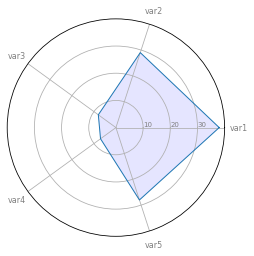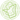A radar chart displays the value of several numerical variables for one or several entities. This post aims to show how to draw a basic radar chart using the basic functions of matplotlib.

In order to build a basic radar chart, we can use the basic functions of matplotlib. Here is an example of a simple one, displaying the values of 5 variables for only one individual. The input data is a pandas data frame where each line represents an individual, and each column a variable. See graph #391 and #392 to see how to represent several individuals.

``````# Libraries
import matplotlib.pyplot as plt
import pandas as pd
from math import pi

# Set data
df = pd.DataFrame({
'group': ['A','B','C','D'],
'var1': [38, 1.5, 30, 4],
'var2': [29, 10, 9, 34],
'var3': [8, 39, 23, 24],
'var4': [7, 31, 33, 14],
'var5': [28, 15, 32, 14]
})

# number of variable
categories=list(df)[1:]
N = len(categories)

# We are going to plot the first line of the data frame.
# But we need to repeat the first value to close the circular graph:
values=df.loc.drop('group').values.flatten().tolist()
values += values[:1]
values

# What will be the angle of each axis in the plot? (we divide the plot / number of variable)
angles = [n / float(N) * 2 * pi for n in range(N)]
angles += angles[:1]

# Initialise the spider plot
ax = plt.subplot(111, polar=True)

# Draw one axe per variable + add labels
plt.xticks(angles[:-1], categories, color='grey', size=8)

# Draw ylabels
ax.set_rlabel_position(0)
plt.yticks([10,20,30], ["10","20","30"], color="grey", size=7)
plt.ylim(0,40)

# Plot data
ax.plot(angles, values, linewidth=1, linestyle='solid')

# Fill area
ax.fill(angles, values, 'b', alpha=0.1)

# Show the graph
plt.show()``````## Contact & Edit

👋 This document is a work by Yan Holtz. Any feedback is highly encouraged. You can fill an issue on Github, drop me a message onTwitter, or send an email pasting `yan.holtz.data` with `gmail.com`.